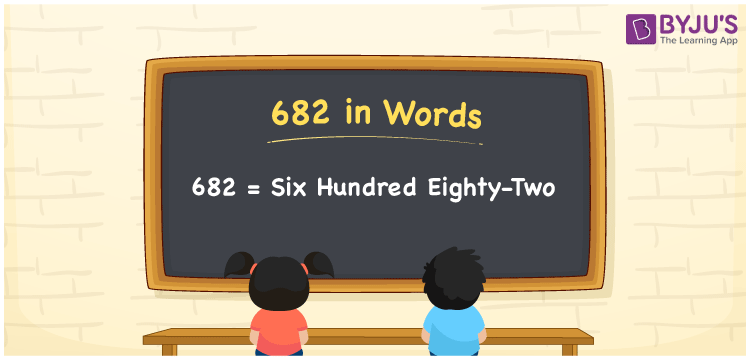# 682 in Words

We can write 682 in words as Six hundred eighty-two. That means Six hundred eighty-two is the word form or number name of the numeral 682. And this is used to express, spell or convey the number verbally to others. If you spent Rs. 682 for buying a textbook, then you can say, “I spent Six hundred eighty-two rupees for buying the textbook”. In the same way, we can use the word form of numbers in general conversations.

 682 in words Six hundred eighty-two Six hundred eighty-two in Numbers 682

## 682 in English Words

We generally use the English alphabet to write numbers in words. Thus, we spell 682 in English words as “Six hundred eighty-two”.## How to Write 682 in Words?

In this section, you will learn how to write the number 682 in words with the help of a place value chart given below.

 Hundreds Tens Ones 6 8 2

Here, ones = 2, tens = 8, hundreds = 6

These numbers can be written as:

6 × Hundred + 8 × Ten + 2 × One

= 6 × 100 + 8 × 10 + 2 × 1

= 600 + 80 + 2

= Six hundred + Eighty + Two

= Six hundred eighty-two

Hence, 682 in words is written as Six hundred eighty-two.

As we know, 682 is a natural number that precedes 683 and succeeds 681.

682 in words – Six hundred eighty-two

Is 682 an odd number? – No

Is 682 an even number? – Yes

Is 682 a prime number? – No

Is 682 a composite number? – Yes

Is 682 a perfect square number? – No

Is 682 a perfect cube number? – No

## Frequently Asked Questions on 682 in Words

Q1

### How do you write 682 in words?

We can write the number 682 in words as Six hundred eighty-two.
Q2

### How do you write Rs. 682 in words on a cheque?

On a cheque, we generally write Rs. 682 in words as “Six hundred eighty-two rupees only”.
Q3

### Express the number 682.50 in words.

The decimal number 682.50 is expressed in words as: 682.50 = Six hundred eighty-two and five-tenths (or) 682.50 = Six hundred eighty-two point five zero